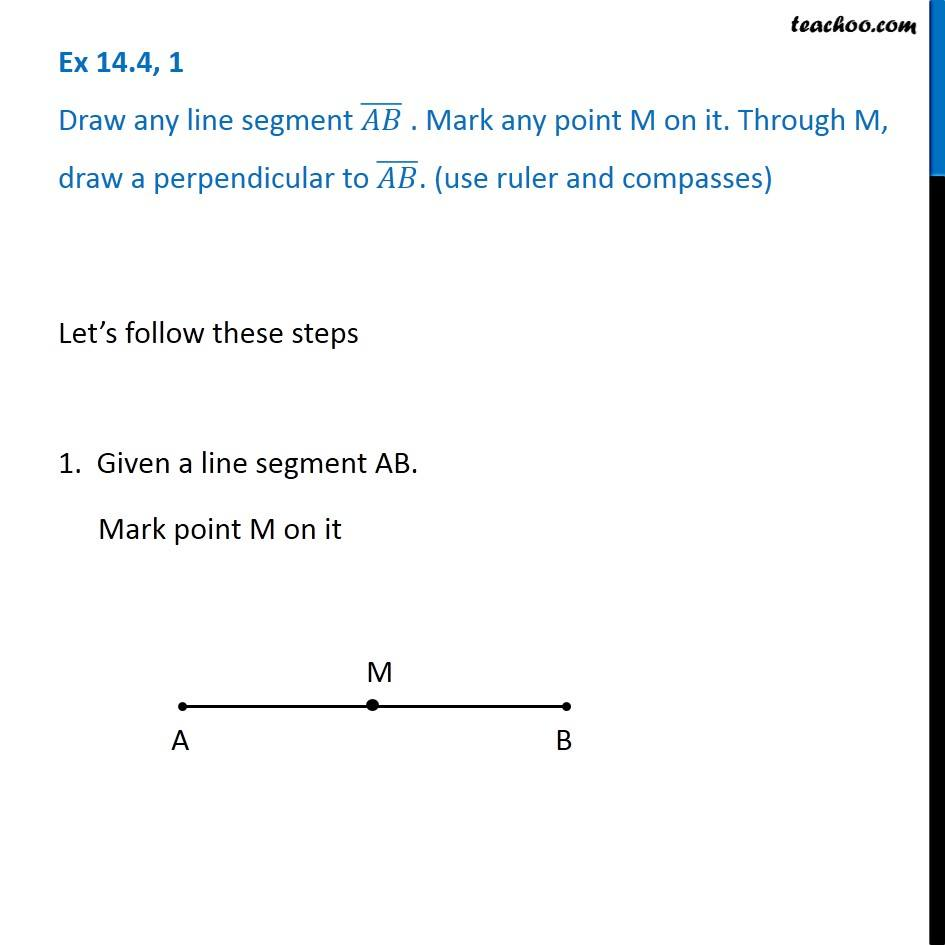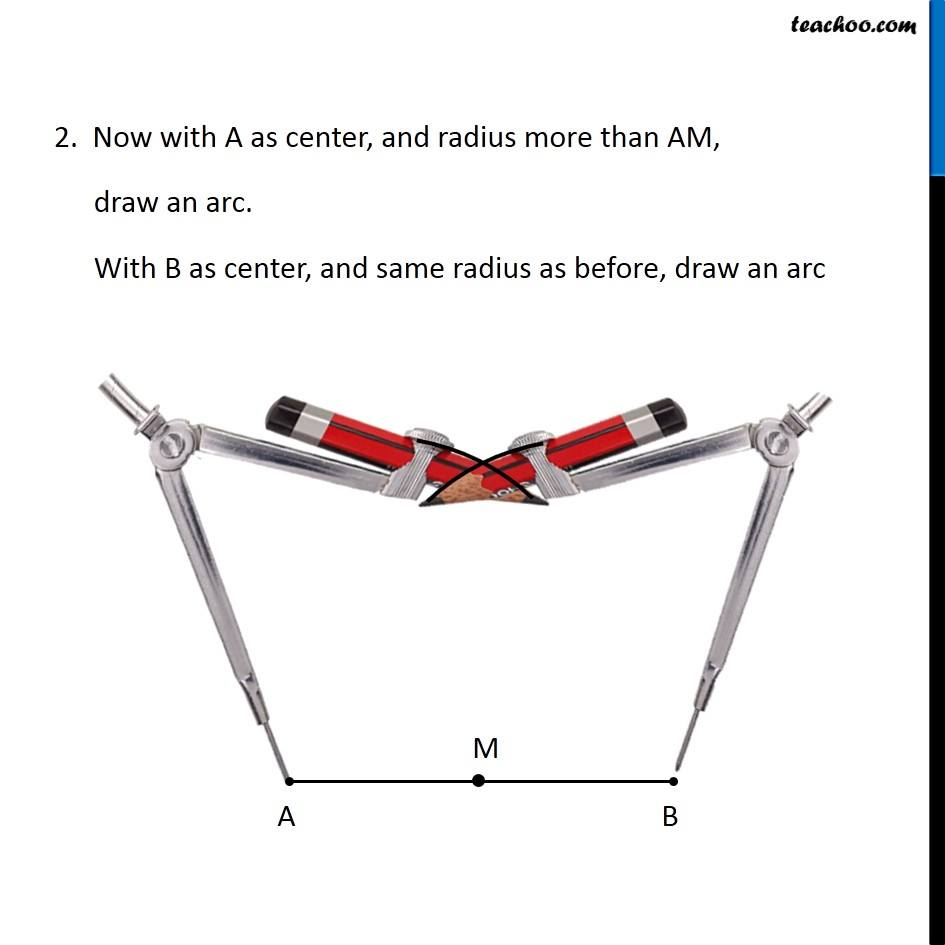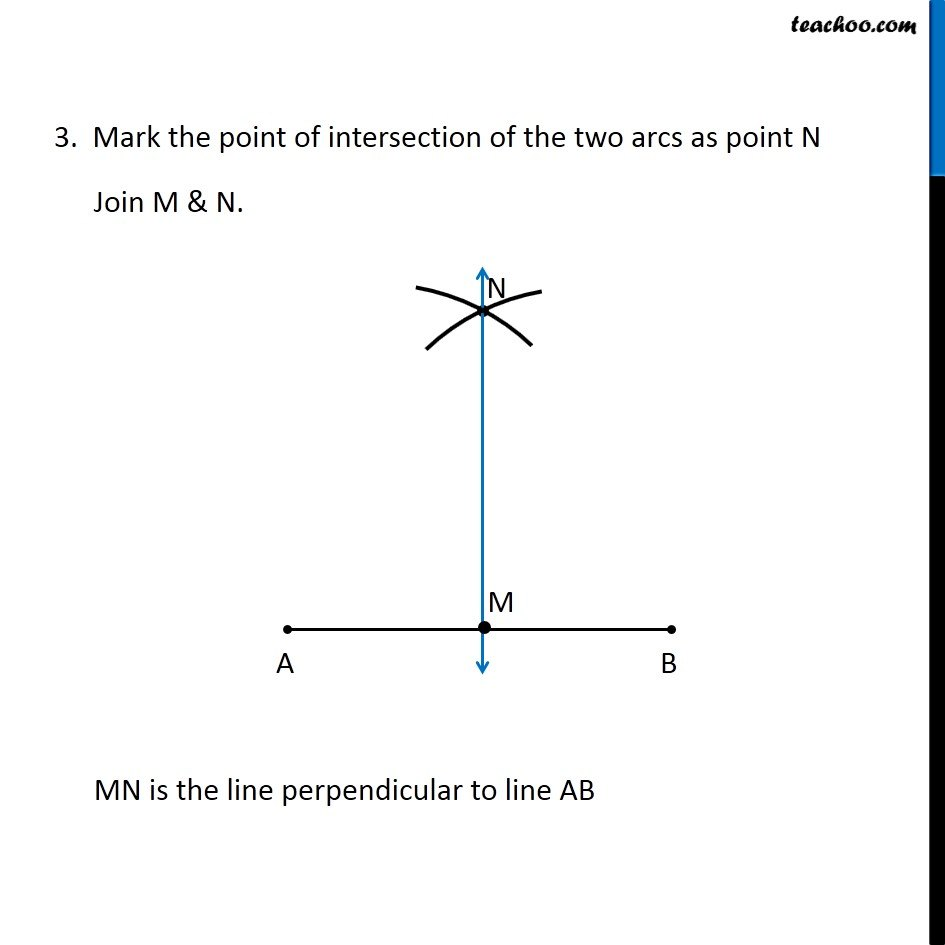Subscribe to our Youtube Channel - https://you.tube/teachoo

1. Chapter 14 Class 6 Practical Geometry
2. Concept wise
3. Perpendicular to a line through a point on it

Transcript

Ex 14.4, 1 Draw any line segment (𝐴𝐵) ̅ . Mark any point M on it. Through M, draw a perpendicular to (𝐴𝐵) ̅. (use ruler and compasses) Let’s follow these steps 1. Given a line segment AB. Mark point M on it 2. Now with A as center, and radius more than AM, draw an arc. With B as center, and same radius as before, draw an arc 3. Mark the point of intersection of the two arcs as point N Join M & N. MN is the line perpendicular to line AB

Perpendicular to a line through a point on it